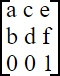#SVG Matrix Transform Example Code by Stephen Bucaro

SVG Matrix Example Code

```<svg width="240" height="120" version="1.1" xmlns="http://www.w3.org/2000/svg" style="border-style:solid;">
<rect x="20" y="20" width="50" height="50" stroke="#000" fill="red" /">
<rect x="20" y="20" width="50" height="50" stroke="#000" fill="green" transform="matrix(1,0,0,1,100,20)" /">
</svg">
```

The matrix transform is the mother of all SVG matrices. By that I mean all the other 2D transformations can be performed with the matrix transform. 2D transformations use a 3x3 matrix that looks like this:2D transformations also use a 1x3 matrix also know as a vector. A 2D vector takes the form [x y 1]. The third value in the matrix is always 1 because in 2D we have no third direction. To use the SVG matrix transform you need provide only the first 6 values of the matrix as parameters. The syntax of the matrix transform is shown below.

transform = "matrix(a,b,c,d,e,f)"

To use the SVG matrix transform you need to be familiar with matrix algebra, which involves coordinate geometry, vector algebra, and trigonometry. That's a steep learning curve and it might be easier to achieve the same thing by stacking the other transforms (translate, scale, rotate, and so on). The SVG matrix transformation example shown above performs a simple translate function.